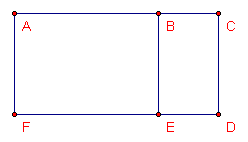# Proportional Rectangles

Is the relationship between the length and the width of similar rectangles measuring 2 units by 1 unit, 4 units by 2 units, and 6 units by 3 units proportional?Extension
Construct two rectangles so that the corresponding sides are proportional. This construction should involve the smaller rectangle sharing a side with the larger rectangle (CD), and two of the sides of the smaller rectangle lie on two of the sides of the larger rectangle. Explain your construction and verify that each pair of sides is proportional.